This topic describes the algorithm used by AutoML for automatic parameter tuning and how to use AutoML.

## Procedure

1. Log on to the Machine Learning Platform for AI (PAI) console and go to the Algorithm Platform tab.
2. In the left-side navigation pane, click Experiments and select an experiment.

The Air Quality Prediction experiment is used in this example.

3. On the tab that appears, choose Auto ML > Auto Parameter Tuning.
4. In the Auto Parameter Tuning dialog box, set the Select Algorithm parameter and click Next.Note If multiple algorithms are used in an experiment, select only one algorithm.
5. In the Configure Parameter Tuning step, set the Parameter Tuning Method parameter and click Next.
Alibaba Cloud PAI provides the following tuning methods:
• EVOLUTIONARY_OPTIMIZER
1. Randomly select A parameter candidate sets. A indicates the value of Exploration Samples.
2. Use the N parameter candidate sets that have high evaluation metrics as the parameter candidate sets for the next iteration.
3. Continue the exploration within R times as the standard deviation range around these parameters to explore new parameter sets. The new parameter sets replace the last (A - N) parameter sets by evaluation metric in the previous round. R indicates the value of Convergence Coefficient.
4. Iterate the exploration for M rounds based on the preceding logic until the optimal parameter set is obtained. M indicates the value of Explorations.

Based on the preceding principle, the final number of models is calculated by using the following formula: A + (A - N) × M.

Notice The first value of N is defined as A/2 - 1, and subsequent values of N are N/2-1 (rounded up).• Data Splitting Ratio: the ratio of training sets to evaluation sets. Input data sources are divided into training and evaluation sets. The value 0.7 indicates that 70% of the data is used for model training and 30% for evaluation.
• Exploration Samples: the number of parameter sets for each iteration. A high value indicates high accuracy and large calculation. This parameter must be set to a positive integer in the range of 5 to 30.
• Explorations: the number of iterations. A high value indicates high accuracy and large calculation. This parameter must be set to a positive integer in the range of 1 to 10.
• Convergence Coefficient: used to tune exploration ranges. A small value indicates fast convergence. However, optimal parameters may be missed. This parameter must be set to a value in the range of 0.1 to 1.
• Custom Range: You must enter the tuning range for each parameter. If a parameter range is not configured, the default range is used, and this parameter is not included in automatic parameter tuning.
• RANDOM_SEARCH

1. Randomly select a value for each parameter within the parameter range.
2. Enter random values into a set of parameters for model training.
3. Perform M rounds and sort the output models. M indicates the value of Explorations.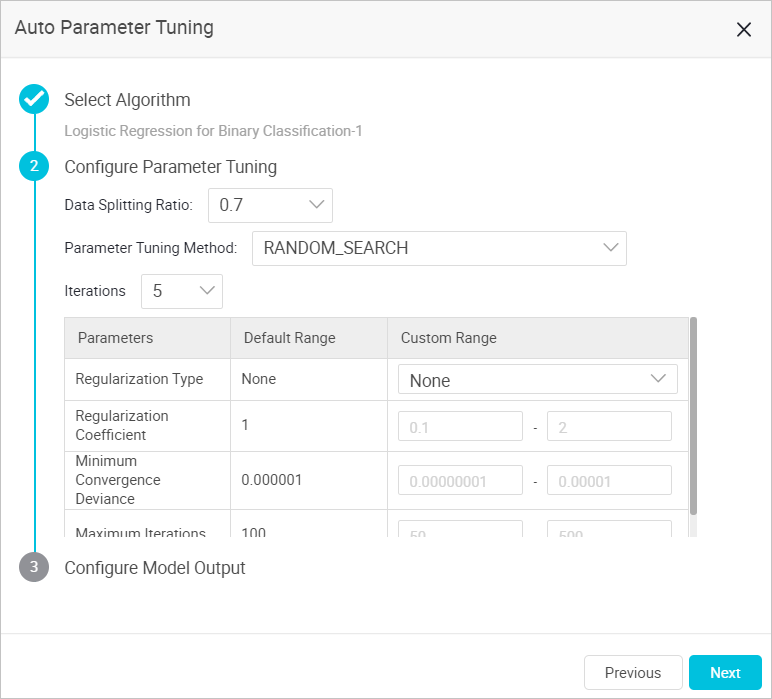• Iterations: the number of searches in the configured range. This parameter must be set to a positive integer in the range of 2 to 50.
• Data Splitting Ratio: the ratio of training sets to evaluation sets. Input data sources are divided into training and evaluation sets. The value 0.7 indicates that 70% of data is used for model training and 30% for evaluation.
• Custom Range: You must enter the tuning range for each parameter. If a parameter range is not configured, the default range is used, and this parameter is not included in automatic parameter tuning.
• GRID_SEARCH

1. Split the value range of each parameter into N segments. N indicates the value of Grids.
2. Randomly select a value from the N segments. Assume that M parameters exist. N^M parameter sets can be combined.
3. Generate a total of N^M models based on the N^M parameter sets. Then, sort the models.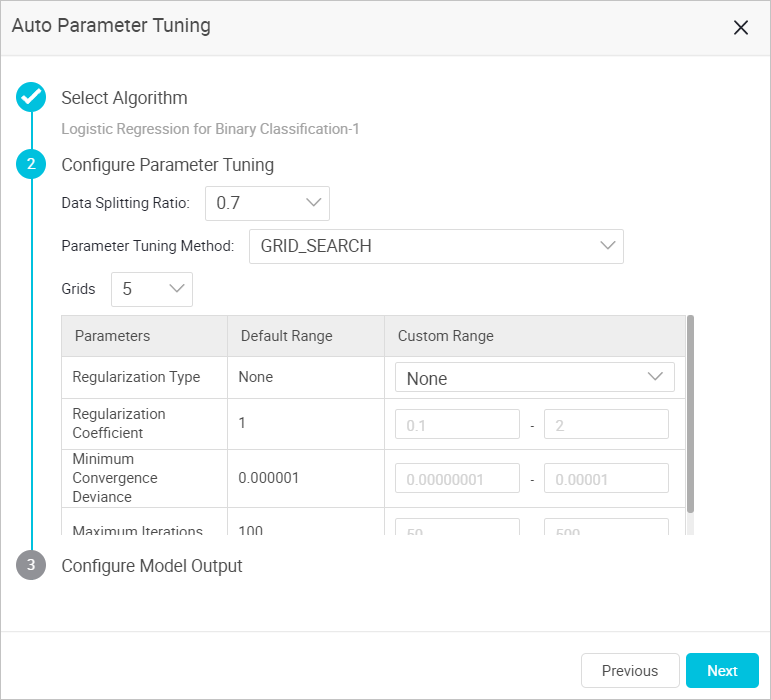• Grids: the number of split grids. This parameter must be set to a positive integer in the range of 2 to 10.
• Data Splitting Ratio: the ratio of training sets to evaluation sets. Input data sources are divided into training and evaluation sets. The value 0.7 indicates that 70% of data is used for model training and 30% for evaluation.
• Custom Range: You must enter the tuning range for each parameter. If a parameter range is not configured, the default range is used, and this parameter is not included in automatic parameter tuning.
• UserDefine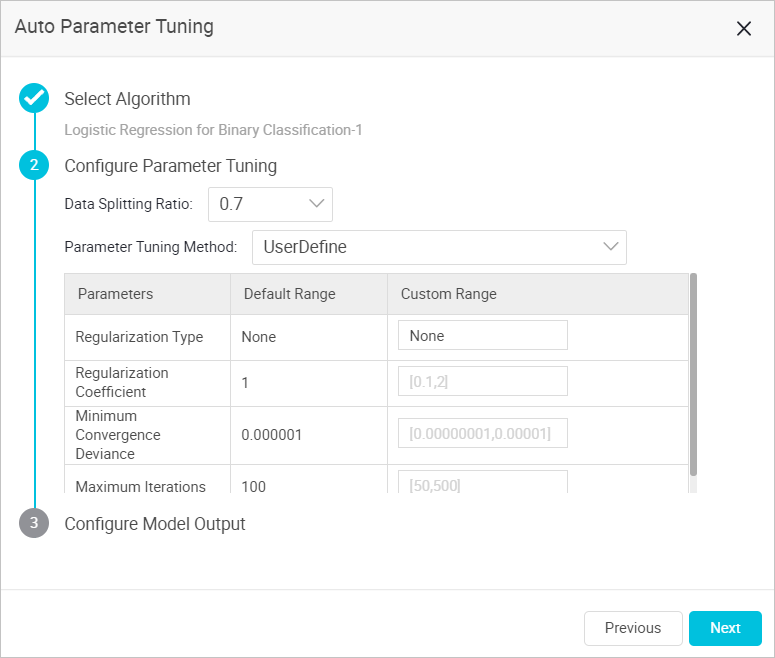Custom Range: The system calculates the score based on all combinations of your parameter values. If the ranges are not configured, the default ranges of parameters are used.

Note In PAI V2.0, the number of parameter tuning algorithms increases from 4 to 7. The following table describes the algorithms.
Algorithm Description
GAUSE This algorithm indicates Gaussian processes. Gaussian processes are examples of Bayesian nonparametric models and are widely used for hyperparameter tuning. Gaussian processes allow the system to observe hyperparameter configuration performance to fit the proxy model, and strengthen decision-making by using model prediction. This way, the system can purposefully select the appropriate hyperparameter results from a limited number of attempts.
SAMPLE This algorithm is developed by the PAI team and DAMO Academy. If large amounts of data are processed, this algorithm allows the system to estimate the final result that a set of hyperparameters can obtain based on part of the data. The SAMPLE algorithm, in combination with the population-based training (PBT) algorithm, allows the system to gradually improve the sampling ratio with the increase in the number of hyperparameters. This way, broader and faster explorations can be performed.
EVOLUTIONARY_OPTIMIZER This algorithm is developed by the PAI team based on the techniques of the PBT algorithm. In the EVOLUTIONARY_OPTIMIZER algorithm logic, parameter tuning is considered a multi-round iteration to progressively explore an optimal solution. The Exploration Samples parameter indicates the number of samples in each iteration. The Explorations parameter indicates the number of iterations. The Convergence Coefficient parameter indicates the step size of each iteration. The EVOLUTIONARY_OPTIMIZER algorithm allows the system to discard the exploration samples that have unsatisfactory results after each iteration. Then, the system expands exploration samples in the set of exploration samples that have satisfactory results to form the sample set for the next iteration. This process is repeated until all the iterations are completed.
PBT PBT is an evolutionary algorithm based on the concept of population. In the PBT algorithm logic, hyperparameter configurations are considered a population and the search process is considered a dynamic environment. In iterations, this algorithm allows the system to filter hyperparameter configurations based on the principle of survival of the fittest. This way, the hyperparameter configurations with the best performance can be obtained. This algorithm is simple and adapts to different data structures to help you obtain optimal results in deep learning model training.
GRID_SEARCH This algorithm allows the system to equally split the parameters that are used in parameter tuning into several sets based on a specific ratio. Then, the system randomly combines the split parameter sets to generate parameter candidate sets for calculation and comparison.
RANDOM_SEARCH The RANDOM_SEARCH algorithm allows the system to randomly obtain and combine samples from each parameter space to form parameter candidate sets for calculation and comparison.
UserDefine Users define parameter sets.
6. In the Configure Model Output step, configure model output parameters and click Next.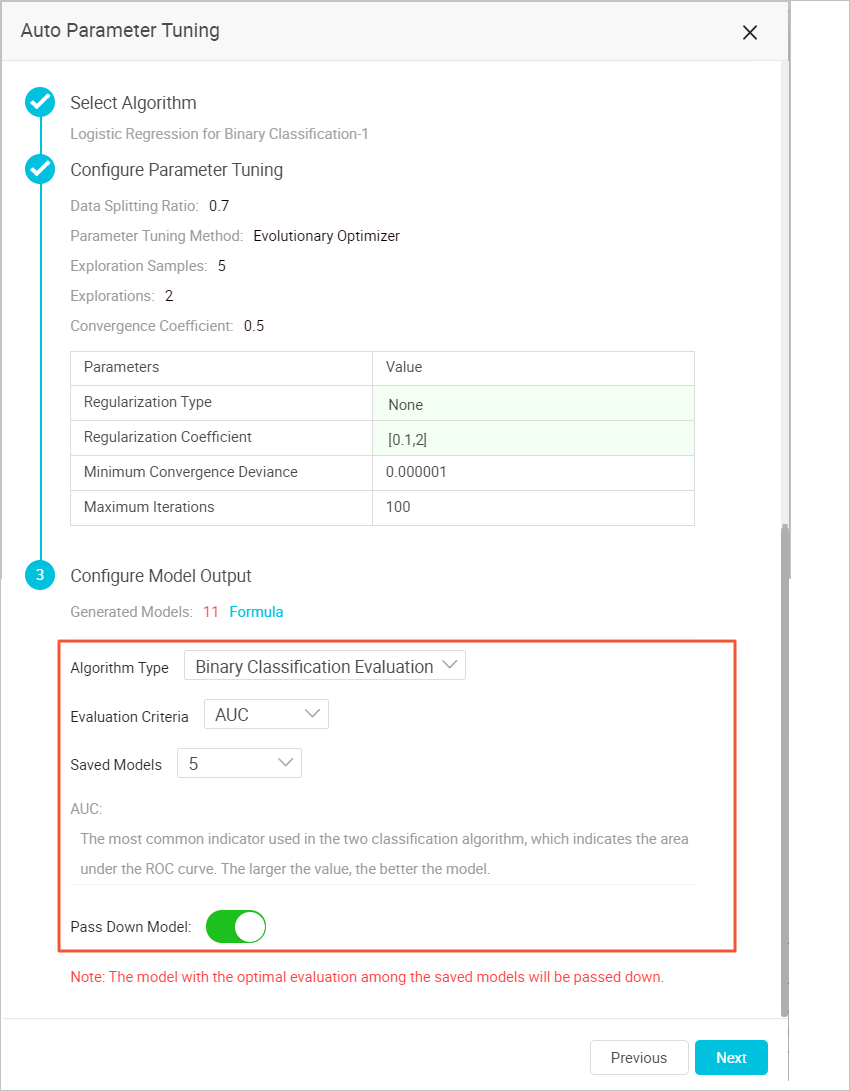• Evaluation Criteria: Valid values are AUC, F1-score, Precision, and Recall.
• Saved Models: Valid values are 1, 2, 3, 4, and 5. The system ranks models based on the value of the Evaluation Criteria parameter that you set and saves the top-ranked models based on the value of the Saved Models parameter.
• Pass Down Model: This switch is turned on by default. If the switch is turned off, the model generated by the default parameters of the current component are passed down to the node of the subsequent component. If the switch is turned on, the optimal model generated by automatic parameter tuning is passed down to the node of the subsequent component.
7. After the configuration, click Run in the upper-left corner of the canvas.
After the preceding configuration is completed, the Auto ML switch of the related algorithm is turned on. You can turn the switch on or off as needed.
8. Optional:Right-click the model and select Edit AutoML parameters to modify AutoML parameters.

## Result

Model output:

1. During parameter tuning, right-click the model component and select Parameter Tuning Details.
2. In the AutoML-Parameter Tuning Details dialog box, click the Metrics tab to view the tuning progress and the running status of each model.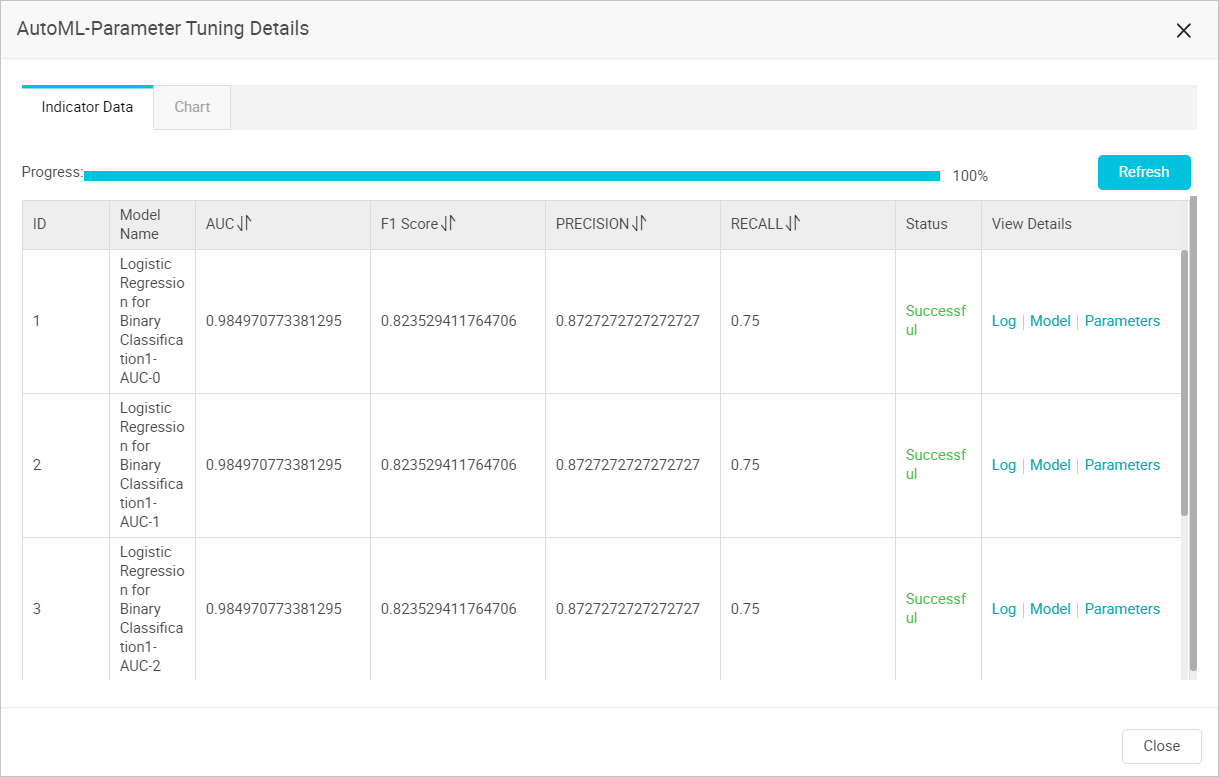3. Sort candidate models based on metrics. The metrics include AUC, F1-score, Accuracy, and Recall Rate.
4. In the View Details column, click Log or Parameter to view the logs and parameters of each candidate model.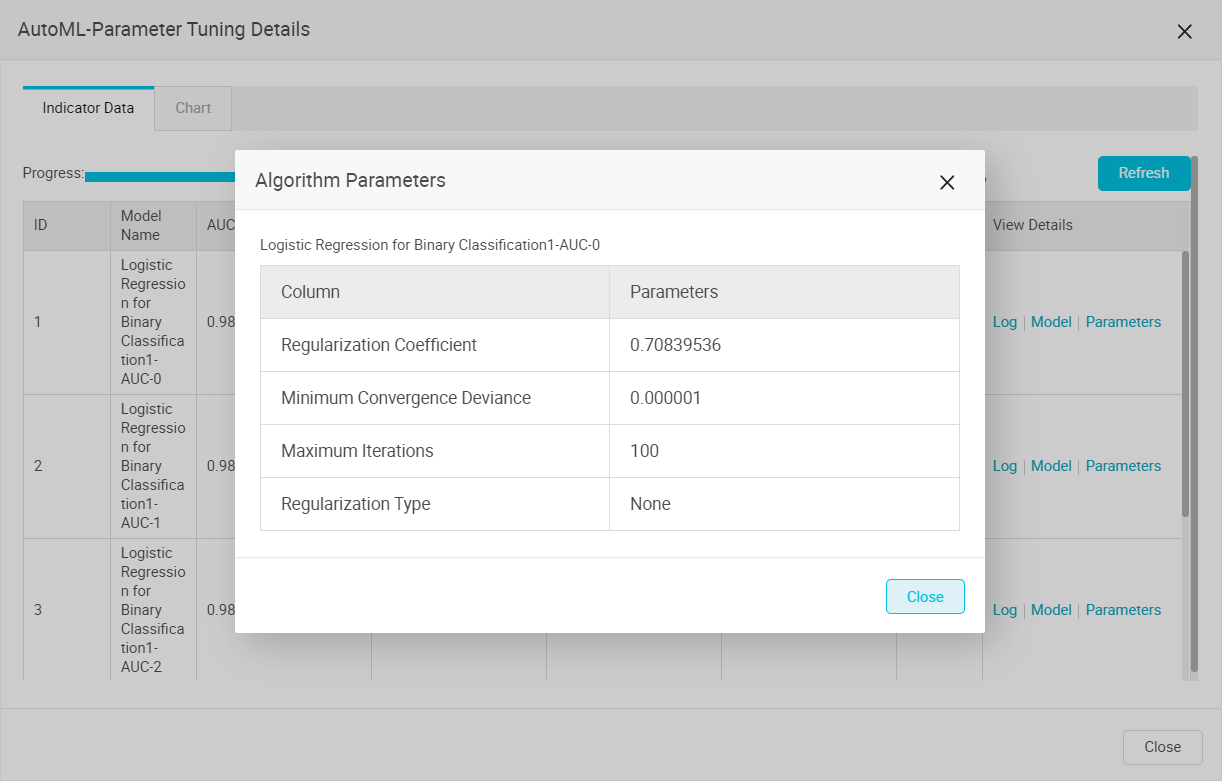Display of parameter tuning effect

You can view the growth trend of the evaluation metrics of updated parameters in the Hyperparameter Iteration Result Comparison chart.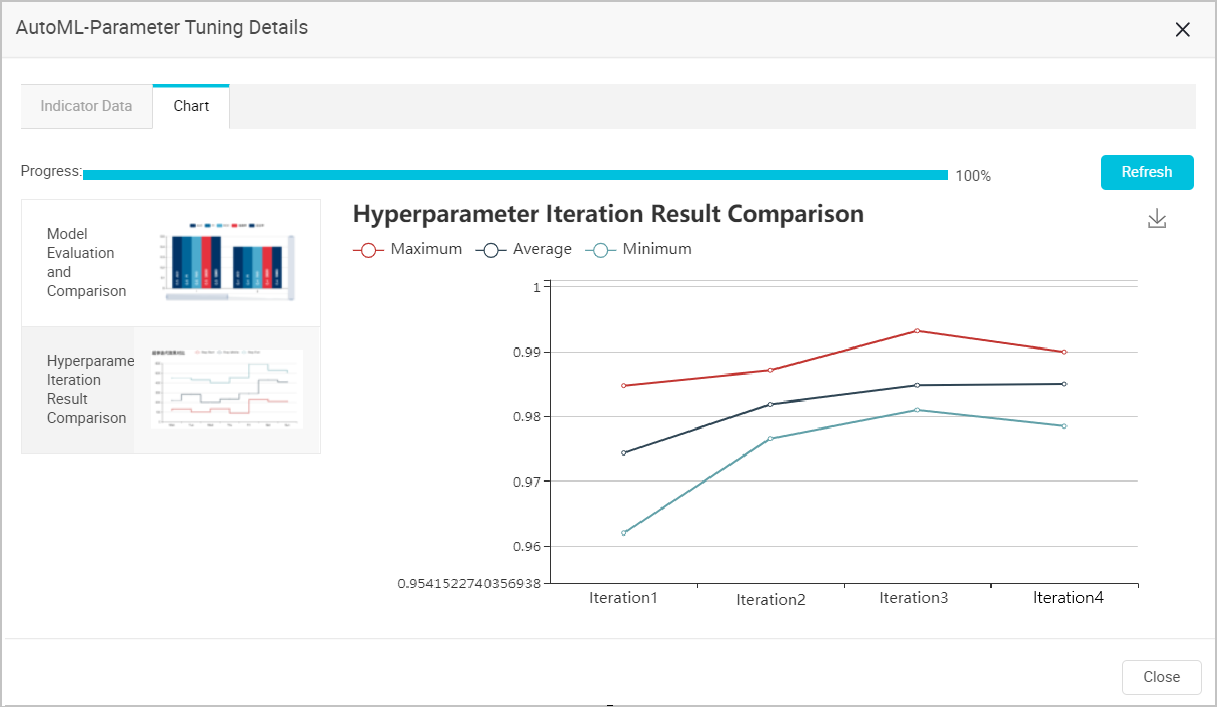Model storage:

1. In the left-side navigation pane of the Algorithm Platform tab, click Models.
2. Click Experiment Models.
3. Click the required experiment folder to view the model saved by using AutoML.